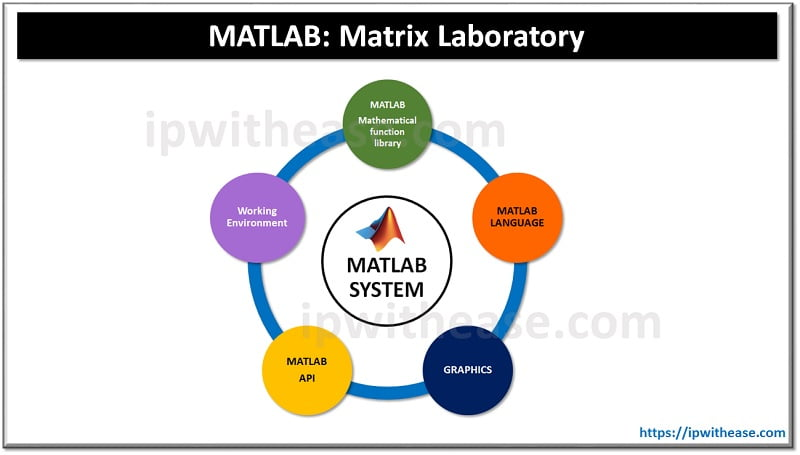# Introduction to MATLAB

AS a software developer you should have come across the name MATLAB. Millions of engineers and scientists use MATLAB for deep learning or machine learning.

Is this your first time hearing about it? Are you wondering what MATLAB means? How it started and how it is now used in the industries.

Don’t worry; in this article, you will get a proper introduction to MATLAB and some basic functions of MATLAB. Here are the contents discussed inside this article.

# What is MATLAB?

MATLAB refers to the Matrix Laboratory. It is a software package used by engineers and programmers to do high-performance mathematical computation and for designing applications.

The main advantage of MATLAB is the MATLAB language, which is a matrix-based language that can help with large mathematical computation, graphical visualization, and animation. It is a Multiparadigm, so it works on different types of programming platforms, such as the functional, object-oriented, and visual environment.

MATLAB is not restricted to a particular field it is used in all the fields it can be in deep learning, robotics, machine learning, finance, etc… And learning MATLAB is very easy and there is always an active help center to guide you.

## History of the MATLAB

The creation of MATLAB started in the 1970s by Cleve Moler. Cleve wanted to help his students to use LINPACK (Linear System Package) and EISPACK (Eigen System Package). LINPACK and EISPACK are the software libraries that are written on FORTRAN.

He wanted to help his students to learn it without knowing FORTRAN so he created MATLAB. Then in 1984 by joining hands with Steve Bangert and Jack Little he rewrote the program in the C language and founded Math Works.

## What are the uses of MATLAB?

MATLAB comes with various built-in functions. Here are the major uses of MATLAB.

• Math and Computations
• Scientific and Engineering Graphics
• Modeling, rendering, animation, and for other types of simulations
• Application and development and designing Graphical User Interface (GUI)
• Algorithm developments
• Data analysis, exploration, and prototyping.

The special thing about MATLAB is it is an interactive system whose basic element is an array without the dimensioning needs. This helps to solve complex mathematical problems especially the ones with matrix and vector formulation.

It can be written in a scalar non-interactive language such as C or FORTRAN. MATLAB has evolved over the years and developed by the voluntary contribution of the different engineers. It features a package of application-specific solutions called toolboxes.

The toolboxes allow you to learn and apply specialized technology or functions called M-files to solve particular classes of problems. MATLAB toolboxes are widely used in the following areas: signal processing, control systems, neural networks, wavelets, simulation, and fuzzy logic, etc…

## Five Main Parts of the MATLAB System:The whole MATLAB system can be divided into five main themes as follow –

### Graphics Handling

The MATLAB graphics system includes the high-level commands for both two and three Dimensional DATA Visualization. You can fully customize the data appearance with few level commands. Using this you create a simple Graphical User Interface on your MATLAB applications.

### Mathematical Functional Library

MATLAB’s mathematical functional library contains a vast collection of algorithms ranging from elementary computational ones like addition, subtraction to complex ones like matrix inverse, Bessel functions, etc… You can implement these functions into your application without much hassle.

### Working Environment

There is a set of tools that helps you to work as a programmer or user with MATLAB. You can import and export data from your workplace and also manage them. It contains MATLAB desktop, command window, editor, Debugger, workspace reports, and search paths.

### MATLAB Language

As we have seen earlier the MATLAB languages are high-level languages with matrix/array-based functions that have control flow statements, input and output, object-oriented programming, and other types of function and data structures. You can create quick and swift programs and also create large and complex application programs using MATLAB.

### Application Program Interface (API)

This library allows you to write C and FORTRAN programs that interact with MATLAB. This gives the following facilities in calling for routine from MATLAB and dynamic linking, calling MATLAB as a computational engine, and for reading and writing MAT-files.

## Conclusion

In conclusion, MATLAB is a user-friendly language equipped with different functions to help you perform different mathematical functions. If you have any questions in the above article please leave them in the comment section below.

What is Terraform?

Best Linux Operating System (OS)

Tags: